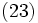# Tour:Join of subgroups

PREVIOUS: Subgroup generated by a subset |UP: Introduction three (beginners) | NEXT: Factsheet three (beginners)
PREREQUISITES: Subgroup generated by a subset
WHAT YOU NEED TO DO:
• Read, and understand, the definition of join of subgroups
• Contrast with something we already saw a little while ago: a union of subgroups is not a subgroup (unless one is contained in the other)
PONDER: Do the operations of join and intersection on subgroups of a group play a similar role as the operations of union and intersection on subsets of a set? Do they obey similar laws (commutativity, associativity, idempotence)? Do they distribute over one another?

## Definition

Suppose$G$ is a group and$H_i$ are subgroups of$G$, where$i \in I$,$I$ an indexing set. The join of the subgroups$H_i$, also called the subgroup generated by the$H_i$s, is defined in the following equivalent way:

• It is the intersection of all subgroups$K \le G$ such that$K$ contains all the$H_i$s
• It is the subgroup generated by the union of the$H_i$s
• It is the set of all elements of$G$ that can be written as a product of elements from the various$H_i$s

The join of finitely many subgroups$H_1, H_2, \ldots, H_n$ is denoted$\langle H_1, H_2, \ldots, H_n \rangle$.

## Related notions

### Union of subgroups

The join of a family of subgroups must contain their union (in fact, it is the subgroup generated by the union). Hence, if the union of a family of subgroups is itself a subgroup, then that equals their join. A particular case of this is when the family of subgroups forms an ascending chain. Another case is where one of the subgroups contains all the others.

Every group is a union of its cyclic subgroups (we take the cyclic subgroup generated by each element). Hence, every group is a join of cyclic subgroups. Further information: every group is a union of cyclic subgroups

A union of two subgroups, neither of which is contained in the other, is not a subgroup. Further information: Union of two subgroups is not a subgroup unless they are comparable

## Examples

• In the group$\mathbb{Z}$ of integers, the join of the subgroup$m\mathbb{Z}$ (multiples of$m$) and the subgroup$n\mathbb{Z}$ (multiples of$n$) is the subgroup generated by the gcd of$m$ and$n$. In other words, an element can be written as$ma + nb, a,b \in \mathbb{Z}$ if and only if it is a multiple of the gcd of$m$ and$n$.
• In the symmetric group on three letters, the join of the subgroup generated by the permutation exchanging the first two letters ($(1 2)$) and the permutation exchanging the second and third letter ($(2 3)$) is the whole group. This is an example of a join that is not the same as the union or the product of the subgroups.
WHAT'S MORE: The relation between join of subgroups, and another notion, product of subgroups, that we haven't yet seen in the tour.

### Product of subgroups

The product of two subgroups$H, K \le G$ is defined as the set of all elements$hk$ where$h \in H, k \in K$. The product depends on the order in which we write the subgroups. In general, the product of two subgroups contains their union and is contained in their join.

In the special case that$HK = KH$, it is a subgroup, and is the same as the join$\langle H, K \rangle$. In such a situation, we say that$H$ and$K$ are permuting subgroups.

This page is part of the Groupprops Guided tour for beginners (Jump to beginning of tour). If you found anything difficult or unclear, make a note of it; it is likely to be resolved by the end of the tour.
PREVIOUS: Subgroup generated by a subset | UP: Introduction three (beginners) | NEXT: Factsheet three (beginners)